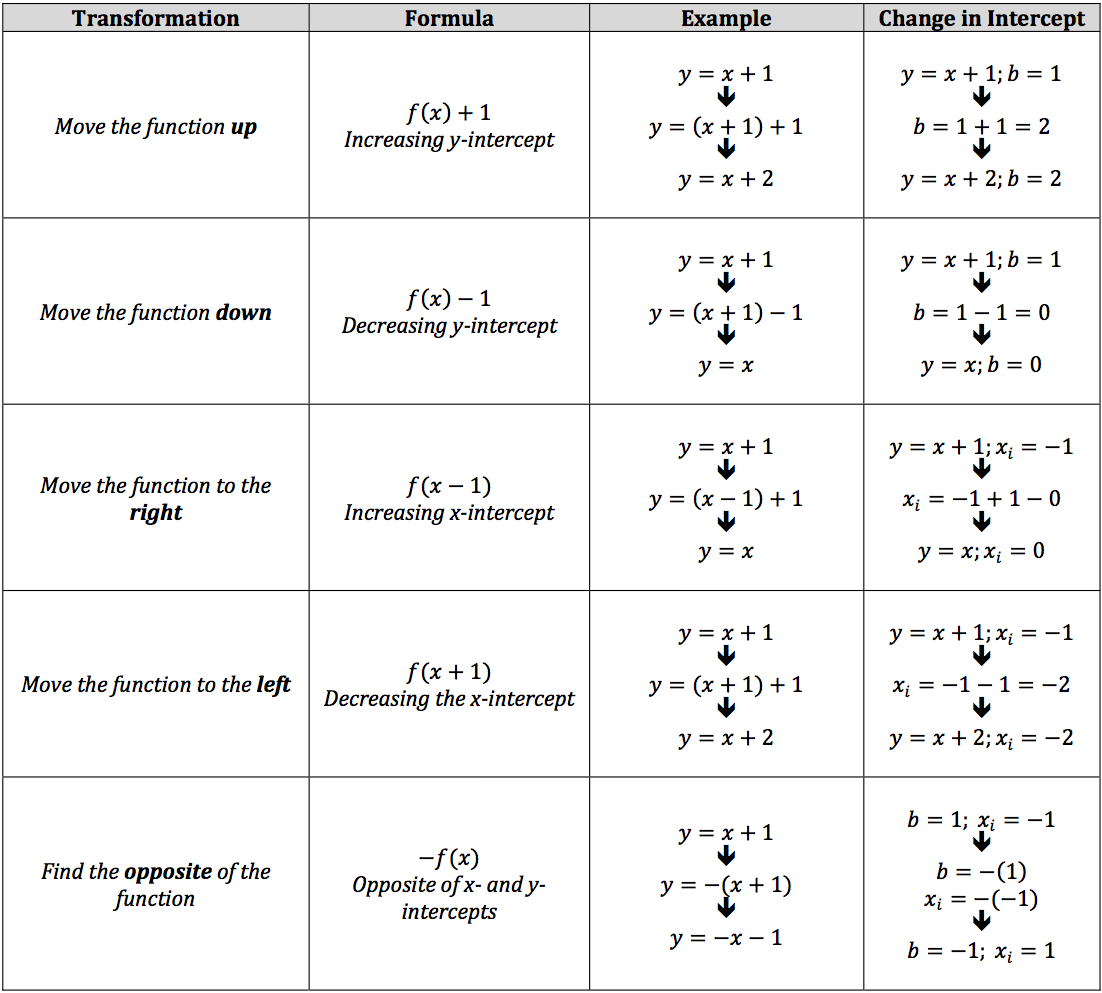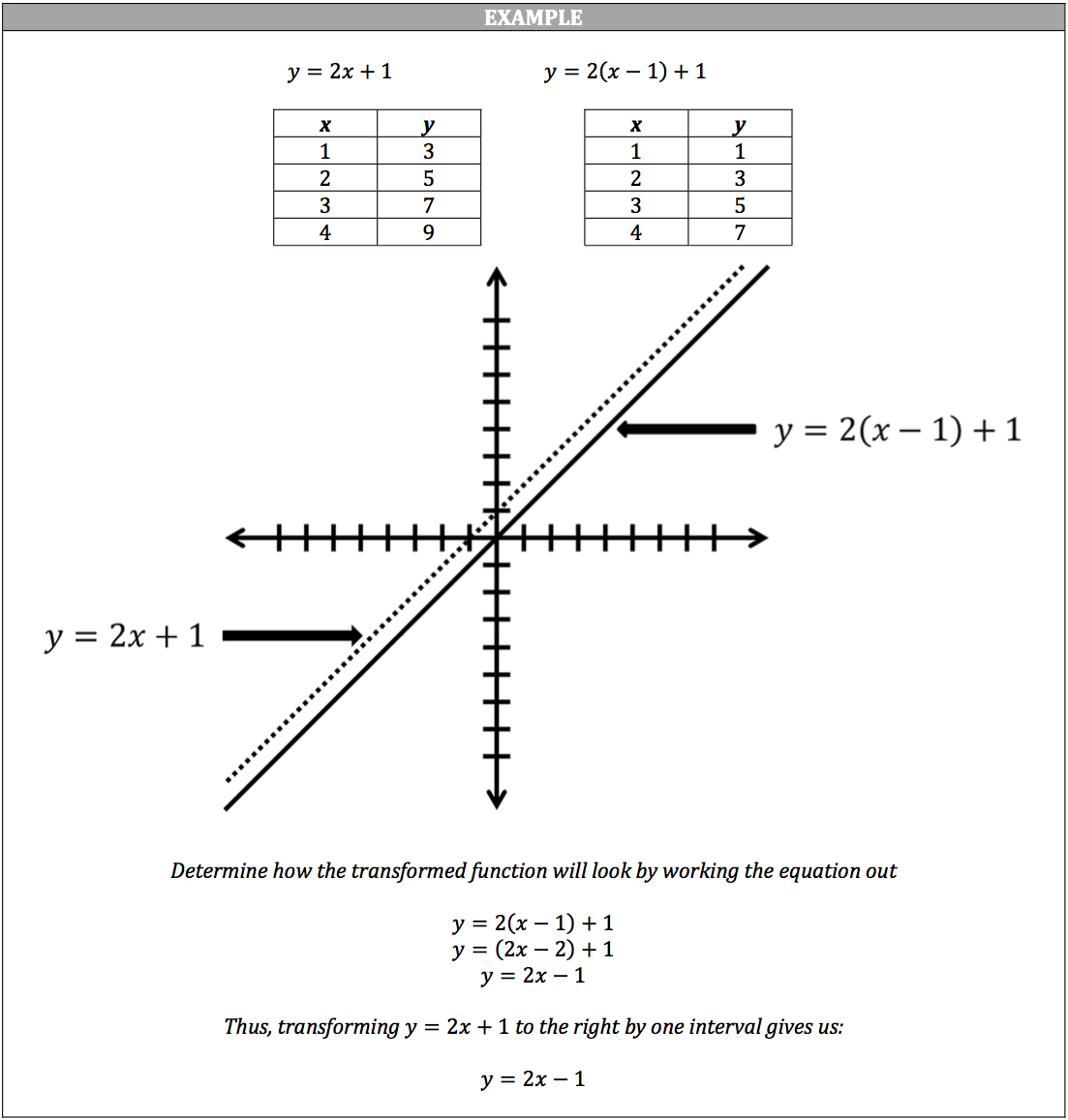A transformation simply refers to moving the graph of a function around:

• If you want to move the line graph of y = 2x + 1 to the left or right, you increase or decrease the input.
• If you want to move the line graph of y = 2x + 1 up or down, you increase or decrease the output.

Normally, the input of 2 for y = 2x + 1 will have the output of 5 and my xy‐coordinate is (2, 5). However, if I decrease the input by 1, y = 2(x – 1) + 1, then my output will be 3 and my xy‐coordinate is (2, 3). The resulting line graph will move to the right by one interval.